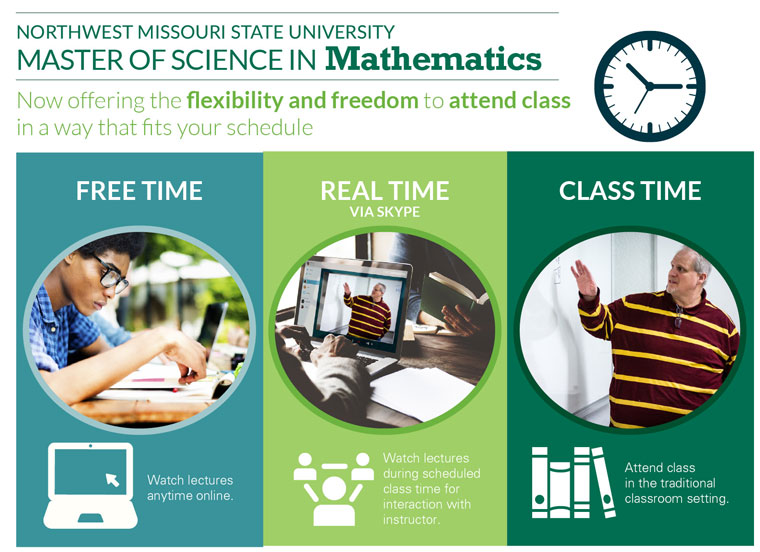# M.S. Mathematics

Also available: Online M.S. in Mathematics | Online M.S.Ed. in Elementary Math• A sequenced program that allows completion of a master’s degree in two years
• Coursework that incorporates a blended format
• A program that offers a variety of courses emphasizing conceptual and practical knowledge
• Elective coursework provides an exploration of newly developing trends in various fields including data mining, new advancements in instructional technology, and mathematics curriculum development
 Every Summer Introduction to Higher Mathematics(3 hours, refresher course) *Possible elective course(3 hours) Fall Odd Analytic Geometry(4 hours) *Possible elective course(3 hours) Spring Even Mathematical Analysis with Applications(4 hours) *Possible elective course(3 hours) Summer Even Combinatorics(4 hours) Research Project(1 hour) Fall Even Number Theory with Applications(4 hours) *Possible elective course(3 hours) Spring Odd Data Analysis(3 hours) *Possible elective course(3 hours) *Degree requires 9 credit hours of electives

## Electives may be chosen from three areas of study:

Research Project

• a supervised graduate-level research project, Math 17-622 or consent of instructor
• The Research Project is equivalent to one-three credit hours and may be repeated for a total of six

Data Mining

• Data mining
• Forecasting
• Computer methods in statistics

Mathematics Education

• Curriculum construction in mathematics
• Rational numbers and ratio and proportion relationships
• Seminar in problem

Call: 660.562.1230 or

Option 1. Students with a minimum undergraduate GPA of 2.75 and composite score of 280 or higher on the verbal and quantitative sections of the GRE General Test will be accepted. The official score must be filed with the Graduate Office prior to admission but no later than the end of the first semester of enrollment. If the score is not submitted by the end of the first academic semester, the student cannot re-enroll until the score is submitted. Students who do not meet the cutoff score of 280 may be accepted on the condition that they maintain a grade point average of at least 3.00 for the first nine graduate hours in mathematics.

Option 2.  Undergraduate mathematics majors within fewer than 30 hours of completing their B.S. in mathematics, with GPA of 2.8 overall and 3.2 in mathematics may apply for a “fast track” admission to the program. Students in this option can complete their B.S. and M.S. in Mathematics in 5 years.

## Comprehensive Examination

The content of the comprehensive examination will be equally divided between those courses offered in the required courses and the list of electives. The degree candidate must pass both portions of the examination.

## Course Descriptions

• Introduction to Higher Mathematics a survey of topics designed to review concepts that include series, differentiation, integration, functions of several variables, maximum and minimum problems, logic, induction, probability, and matrices. (3 credit hours)
• Analytic Geometry — a content course where students will deepen their knowledge and appreciation of geometry. Topics may include transformation geometry, taxicab geometry, parametric equations, polar coordinates, conic sections, and famous theorems in geometry. (4 credit hours)
• Mathematical Analysis with Applications — topics include sequence and series, continuity, differentiability, integration of functions of one real variable and functions of one complex variable. Applications to engineering and modeling problems will be presented. (4 credit hours)
• Combinatorics — an introduction to sets and their properties, functions, finite and infinite sets, and fundamental techniques in enumeration, discrete structures, and algorithms and optimization. (4 credit hours)
• Number Theory with Applications — a standard course in classical number theory. Topics include divisibility, congruence, theory of quadratic residues, and Diophantine analysis. (4 credit hours)
• Data Analysis — a course is designed to explain what data mining is all about and show how to use data mining techniques effectively in practice. It will be a practical, hands-on introduction to the statistical methods based on case-studies. It provides an introduction and encouragement for more advanced work on real big data. Although the mathematics behind the methodologies used will be explained, the focus will be on the practical issues of getting results out of data mining. Some knowledge of statistical modeling, especially regression techniques will be useful. (3 credit hours)